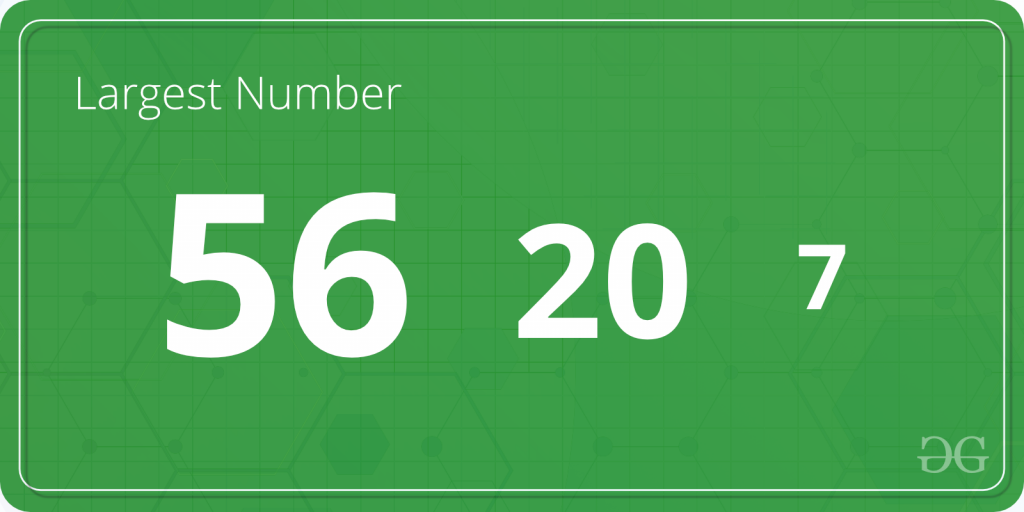# TCS Coding Practice Question | Greatest of 3 Numbers

Given three numbers, the task is to find the greatest of three numbers using Command Line Arguments.Examples:

```Input: A = 2, B = 8, C = 1
Output: 8

Input: A = 231, B = 4751, C = 75821
Output: 75821
```

Approach:

• Since the numbers are entered as Command line Arguments, there is no need for a dedicated input line
• Extract the input numbers from the command line argument
• This extracted numbers will be in String type.
• Convert these numbers into integer type and store it in variables, say A, B and C
• Find the greatest of the numbers as follows:
• Check if A is greater than B.
• If true, then check if A is greater than C.
• If true, print ‘A’ as the greatest number.
• If false, print ‘C’ as the greatest number.
• If false, then check if B is greater than C.
• If true, print ‘B’ as the greatest number.
• If false, print ‘C’ as the greatest number.
• Print or return the greatest numbers

Program:

## C

 `// C program to compute the greatest of three numbers ` `// using command line arguments ` ` `  `#include ` `#include /* atoi */ ` ` `  `// Function to compute the greatest of three numbers ` `int` `greatest(``int` `A, ``int` `B, ``int` `C) ` `{ ` ` `  `    ``if` `(A >= B && A >= C) ` `        ``return` `A; ` ` `  `    ``if` `(B >= A && B >= C) ` `        ``return` `B; ` ` `  `    ``return` `C; ` `} ` ` `  `// Driver code ` `int` `main(``int` `argc, ``char``* argv[]) ` `{ ` ` `  `    ``int` `num1, num2, num3; ` ` `  `    ``// Check if the length of args array is 1 ` `    ``if` `(argc == 1) ` `        ``printf``(``"No command line arguments found.\n"``); ` ` `  `    ``else` `{ ` ` `  `        ``// Get the command line argument and ` `        ``// Convert it from string type to integer type ` `        ``// using function "atoi( argument)" ` `        ``num1 = ``atoi``(argv); ` `        ``num2 = ``atoi``(argv); ` `        ``num3 = ``atoi``(argv); ` ` `  `        ``// Find the greatest and print it ` `        ``printf``(``"%d\n"``, greatest(num1, num2, num3)); ` `    ``} ` `    ``return` `0; ` `} `

## Java

 `// Java program to compute the greatest of three numbers ` `// using command line arguments ` ` `  `class` `GFG { ` ` `  `    ``// Function to compute the greatest of three numbers ` `    ``static` `int` `greatest(``int` `A, ``int` `B, ``int` `C) ` `    ``{ ` ` `  `        ``if` `(A >= B && A >= C) ` `            ``return` `A; ` ` `  `        ``if` `(B >= A && B >= C) ` `            ``return` `B; ` ` `  `        ``return` `C; ` `    ``} ` ` `  `    ``// Driver code ` `    ``public` `static` `void` `main(String[] args) ` `    ``{ ` ` `  `        ``// Check if length of args array is ` `        ``// greater than 0 ` `        ``if` `(args.length > ``0``) { ` ` `  `            ``// Get the command line argument and ` `            ``// Convert it from string type to integer type ` `            ``int` `num1 = Integer.parseInt(args[``0``]); ` `            ``int` `num2 = Integer.parseInt(args[``1``]); ` `            ``int` `num3 = Integer.parseInt(args[``2``]); ` ` `  `            ``// Find the greatest ` `            ``int` `res = greatest(num1, num2, num3); ` ` `  `            ``// Print the greatest ` `            ``System.out.println(res); ` `        ``} ` `        ``else` `            ``System.out.println(``"No command line "` `                               ``+ ``"arguments found."``); ` `    ``} ` `} `

Output:

Attention reader! Don’t stop learning now. Get hold of all the important DSA concepts with the DSA Self Paced Course at a student-friendly price and become industry ready.

My Personal Notes arrow_drop_upContent Manager at LambdaTest

If you like GeeksforGeeks and would like to contribute, you can also write an article using contribute.geeksforgeeks.org or mail your article to contribute@geeksforgeeks.org. See your article appearing on the GeeksforGeeks main page and help other Geeks.

Please Improve this article if you find anything incorrect by clicking on the "Improve Article" button below.

Article Tags :
Practice Tags :

2

Please write to us at contribute@geeksforgeeks.org to report any issue with the above content.